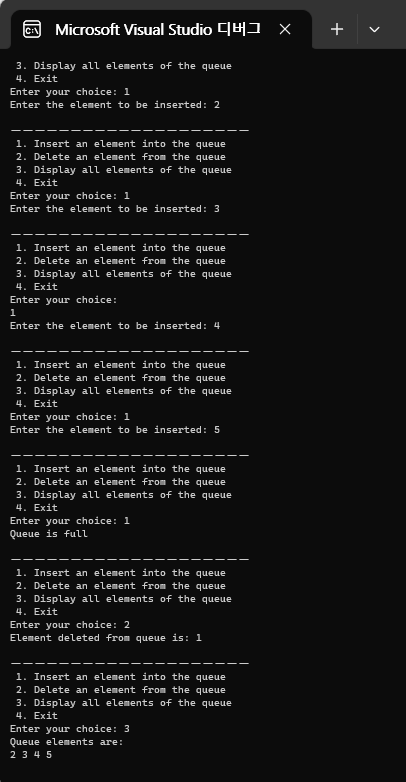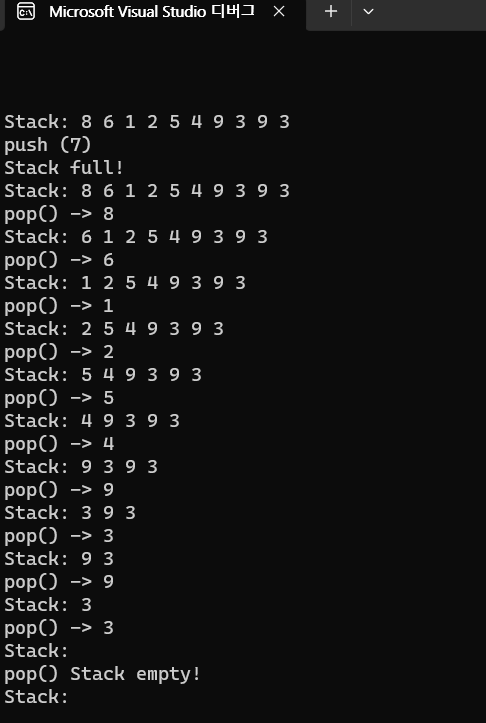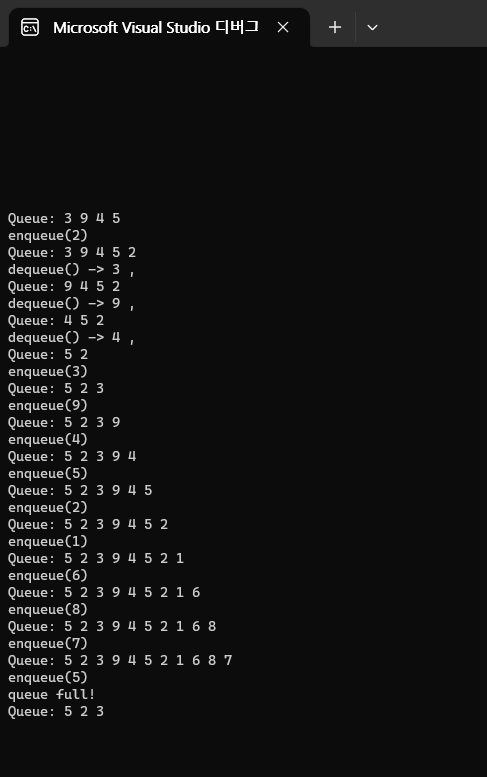# Data Steuctures - Lists : Stacks and Queues 2

119kyh·2023년 3월 21일
0

목록 보기
2/5

# Hw1-1

• Implement a ring buffer with an array of 5
elements that uses buffer overflow.
• Test the program using the sequence of
inserts and deletes

## Source Code

/*
Implement a ring buffer with an array of 5
elements that uses buffer overflow.

Circluar queue using ring buffer (array)

Test the program using the sequence of inserts and deletes
*/
#include<stdio.h>

int main() {
int queue;
int front = -1, rear = -1;
int choice = 0;
int item = 0;

while (choice != 4) {
printf("\nㅡㅡㅡㅡㅡㅡㅡㅡㅡㅡㅡㅡㅡㅡㅡㅡㅡㅡㅡㅡ\n");
printf(" 1. Insert an element into the queue \n");
printf(" 2. Delete an element from the queue \n");
printf(" 3. Display all elements of the queue \n");
printf(" 4. Exit \n");
scanf("%d", &choice);

switch (choice) {
case 1:
if ((front == 0 && rear == 4) || (front == rear + 1)) {
printf("Queue is full \n");
}
else {
if (front == -1) {
front = 0;
}
rear = (rear + 1) % 5;
printf("Enter the element to be inserted: ");
scanf("%d", &item);
queue[rear] = item;
}
break;
case 2:
if (front == -1) {
printf("Queue is empty \n");
}
else {
item = queue[front];
printf("Element deleted from queue is: %d \n", item);
if (front == rear) {
front = -1;
rear = -1;
}
else {
front = (front + 1) % 5;
}
}
break;
case 3:
if (front == -1) {
printf("Queue is empty \n");
break;
}
else {
printf("Queue elements are: \n");
if (front <= rear) {
for (int i = front; i <= rear; i++) {
printf("%d ", queue[i]);
}
printf("\n");
break;
}
else {
for (int i = front; i < 5; i++) {
printf("%d ", queue[i]);
}
for (int i = 0; i <= rear; i++) {
printf("%d ", queue[i]);
}
printf("\n");
break;
}
}
default:
printf("Invalid choice \n");
break;
}
}

return 0;
}# Hw1-2

• Implement and Test a Stack Program, Using a

• The order of insert and delete is the same.
Outputs the current stack or queue contents for each insert or delete)

## Source Code

#include<stdio.h>
#include<stdlib.h>
#define MAX_SIZE 10

struct Node
{
int data;
};

struct Node* top = NULL; // top is empty initially
int n_nodes = 0; // a variable to store number of nodes in stack

int stack_full() {
if (n_nodes == MAX_SIZE) {
return 1;
}
else {
return 0;
}
}

int stack_empty() {
if (n_nodes == 0) {
return 1;
}
else {
return 0;
}
}

void print_stack() {
struct Node* temp = top;
printf("\nStack: ");
while (temp != NULL) {
printf("%d ", temp->data);
}
printf("\n");
}

void push(int x) {
struct Node* temp = (struct Node*)malloc(sizeof(struct Node));
// test if memory is failed
if (temp == NULL) {
printf("Memory allocation is failed.\n");
exit(1);
}
temp->data = x;
top = temp;
n_nodes++;
}

int pop() {
// free a deleted (disconnected) node
struct Node* temp = top;
if (top == NULL) {
printf("Stack is empty.\n");
exit(1);
}
int x = temp->data;
free(temp);
n_nodes--;

return x;
}

// helper function: run a series of pushes
// input arguments: int arr[] <- an array from which input values are taken
// input arguments: int num <- total number of elements to push
void run_pushes(int arr[], int num) {
for (int i = 0; i < num; i++) {
printf("push (%d)", arr[i]);

if (!stack_full()) {
push(arr[i]);
}
else {
printf("\nStack full!");
print_stack();
break;
}
print_stack();
}
}

// helper function: run a series of pops
// input argument: int num <- total number of elements to pop
void run_pops(int num) {
for (int i = 0; i < num; i++) {
printf("pop() ");
if (!stack_empty()) { // if stack is not empty (!1 = 0)
printf("-> %d", pop());
}
else {
printf("Stack empty!");
print_stack();
break;
}
print_stack();
}
}

int main() {
int numbers[] = { 3, 9, 4, 5, 2, 1, 6, 8, 7, 5, 8 };

print_stack();
run_pushes(numbers, 5);
run_pops(3);
run_pushes(numbers, 10);
run_pops(11);

return 0;
}# Hw1-3

• Implement and Test a Queue Program, Using

• The order of insert and delete is the same.
Outputs the current stack or queue contents for each insert or delete)

## Source Code

#include<stdio.h>
#include<stdlib.h>
#define MAX_SIZE 10

struct Node
{
int data;
};

struct Node* front = NULL; // front is empty initially
struct Node* rear = NULL; // rear is empty initially
int n_nodes = 0; // a variable to store number of nodes in queue

int queue_full() {
if (n_nodes == MAX_SIZE) {
return 1;
}
else {
return 0;
}
}
int queue_empty() {
if (n_nodes == 0) {
return 1;
}
else {
return 0;
}
}

void enqueue(int x) {
struct Node* temp = (struct Node*)malloc(sizeof(struct Node));
// test if memory is failed
if (temp == NULL) {
printf("Memory allocation is failed.\n");
exit(1);
}
temp->data = x;
if (front == NULL && rear == NULL) {
front = rear = temp;
return;
}
rear = temp;
n_nodes++;
}

int dequeue() {
// free a deleted (disconnected) node
struct Node* temp = front;
if (front == NULL) {
printf("Queue is empty\n");
return -1;
}
if (front == rear) {
front = rear = NULL;
}
else {

}
int x = temp->data;
free(temp);
n_nodes--;

return x;
}

// helper function: traverse queue from front to rear and print elements
void print_queue() {
struct Node* temp = front;
printf("\nQueue: ");
while (temp != NULL) {
printf("%d ", temp->data);
}
printf("\n");
}

// helper function: run a series of enqueues
// input arguments: int arr[] <- an array from which input values are taken
// input arguments: int num <- total number of elements to push

void run_enqueues(int arr[], int num) {
for (int i = 0; i < num; i++) {
printf("enqueue(%d)", arr[i]);
if (!queue_full()) {
enqueue(arr[i]);
}
else {
printf("\nqueue full!");
print_queue();
break;
}
print_queue();
}
}

// helper function: run a series of pops
// input argument: int num <- total number of elements to pop
void run_dequeues(int num) {
int value;
for (int i = 0; i < num; i++) {
printf("dequeue() ");
if (!queue_empty()) {
value = dequeue();
printf("-> %d , ", value);
}
else {
printf("queue empty! ");
front = rear = NULL;
print_queue();
break;
}
print_queue();
}
}

int main() {
int numbers[] = { 3, 9, 4, 5, 2, 1, 6, 8, 7, 5, 8 };

print_queue();
run_enqueues(numbers, 5);
run_dequeues(3);
run_enqueues(numbers, 10);
run_dequeues(11);
run_enqueues(numbers, 11);

return 0;
}